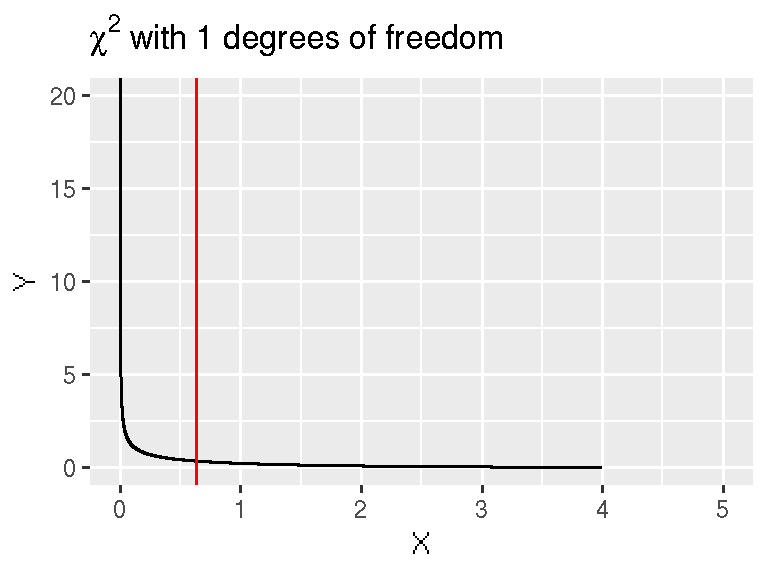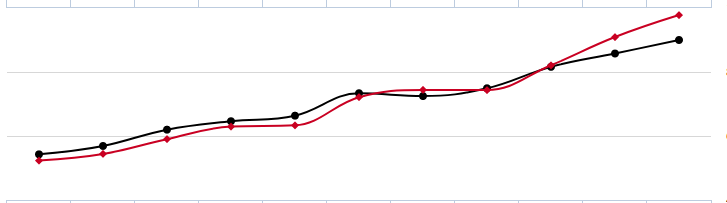2018-10-24

## Associations between categorical variables

• Row x Column table (2 x 2 simplest)
• Categorical data
• Look for association (relationship) between row variable and column variable
• N.B. we have already seen an example of this in the Lady tasting tea experiment

## Should you wear a bicycle helmet?

• In a study, 372 people wearing a helmet received head injuries compared to 267 that were not
• but this does not show the full picture.

## Should you wear a bicycle helmet?

• It turns out that far more people in the study were wearing helmets
• Analysis on the data shows that a much higher proportion of the cyclists not wearing a helmet have a higher proportion of head injuries
                   Head.Injury Other.Injury
Wearing Helmet             372         4715
Not Wearing Helmet         267         1391

## Chi-square test

• E.g. Research question: A trial to assess the effectiveness of a new treatment versus a placebo in reducing tumour size in patients with ovarian cancer.
          Tumour.Did.Not.Shrink Tumour.Did.Shrink
Treatment                    44                40
Placebo                      24                16
• Is there an association between treatment group and tumour shrinkage
• Null hypothesis, $$H_0$$: No association
• Alternative hypothesis, $$H_1$$: Some association

## Chi-square test: calculating expected frequencies

          Tumour.Did.Not.Shrink Tumour.Did.Shrink Total
Treatment                    44                40    84
Placebo                      24                16    40
Total                        68                56    68

$E = \frac{row total \times col total}{overall total}$

• e.g. for row 1, column 1 $\frac{84}{124} \times \frac{68}{124} \times 124 = \frac{84\times68}{124} = 46.1$

## Chi-square test: calculating the chi-square statistic

Observed frequencies:

          Tumour.Did.Not.Shrink Tumour.Did.Shrink
Treatment                    44                40
Placebo                      24                16

Expected frequencies:

          Tumour.Did.Not.Shrink Tumour.Did.Shrink
Treatment                  46.1              37.9
Placebo                    21.9              18.1

$\chi^2_1 = \frac{(44-46.06)^2}{46.06} + \frac{(40-37.94)^2}{37.94} + \frac{(24-21.94)^2}{21.94} + \frac{(16-18.06)^2}{18.06}$

## Chi-square testTest statistic: $${\chi_1}^2$$ = 0.43 df = 1 P-value = 0.43

Do not reject $$H_0$$ (No evidence of an association between treatment group and tumour shrinkage)

## Limitations of the chi-square test

• In general, a Chi-square test is appropriate when:
• at least 80% of the cells have an expected frequency of 5 or greater
• none of the cells have an expected frequency less than 1
• If these conditions aren’t met, Fisher’s exact test should be used.

## Same question, smaller sample size

• e.g. Research question: Is there an association between treatment group and tumour shrinkage?
          Tumour.Did.Not.Shrink Tumour.Did.Shrink Total
Treatment                     8                 3    11
Placebo                       9                 4    13
Total                        17                 7    17
• Null hypothesis: $$H_0$$: No association
• Alternative hypothesis: $$H_1$$: Some association

## Fishers' exact test; results

Expected frequencies:-

          Tumour.Did.Not.Shrink Tumour.Did.Shrink
Treatment                   7.8               3.2
Placebo                     9.2               3.8
• Test statistic: N/A
• P-value 1
• Interpretation: *Do not reject $$H_0$$ (No evidence of an association between treatment group and tumour shrinkage)

## Summary - Categorical variables

• Chi-square test
• Use when we have two categorical variables, each with two or more levels, and our expected frequencies are not too small.
• Fishers exact test
• Use when we have two categorical variables, each with two levels, and our expected frequencies are small.
• (Chi-square test for trend)
• Use when we have two categorical variables, where one or both are naturally ordered and the ordered variable has at least three levels, and our expected frequencies are not too small.
• (McNemar’s test)
• Use when we have two categorical paired variables.

## Summary - Categorical variables

• Turn scientific question to null and alternative hypothesis

• Calculate expected frequencies

• Carry out chi-square or Fishers test if appropriate

## Contingency table practical

• Complete contingency table practical

## Small group Exercise

• Inside the folder mystery-data you will find 8 csv files containing data for analysis
• details are given in the practical
• Each group of 3/4 people will be assigned a dataset to analyse
• On this interactive document, describe how you approached the analysis, what test you used and your conclusions

## Common pitfalls## Common pitfalls

Correlation does not equal causation